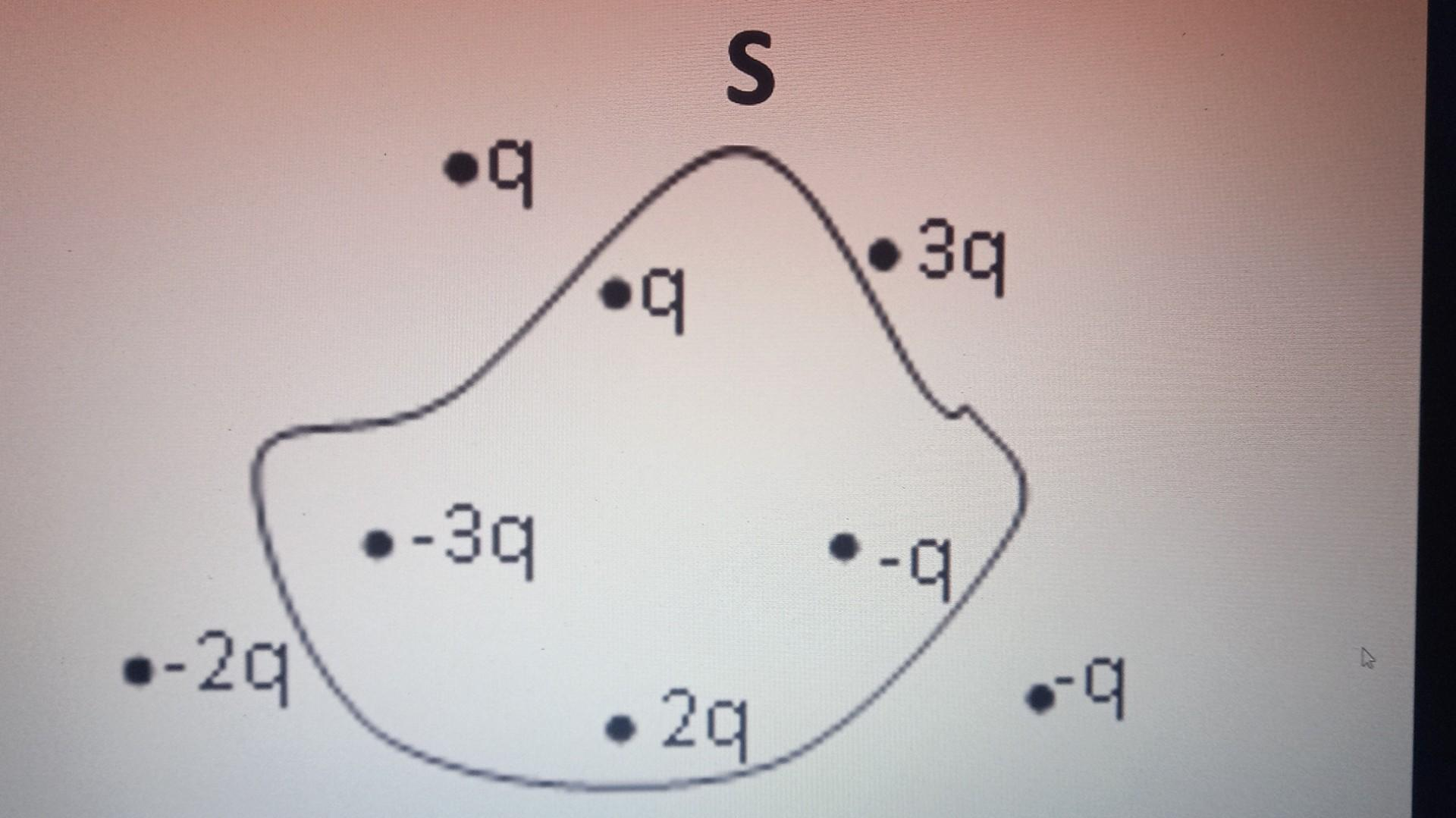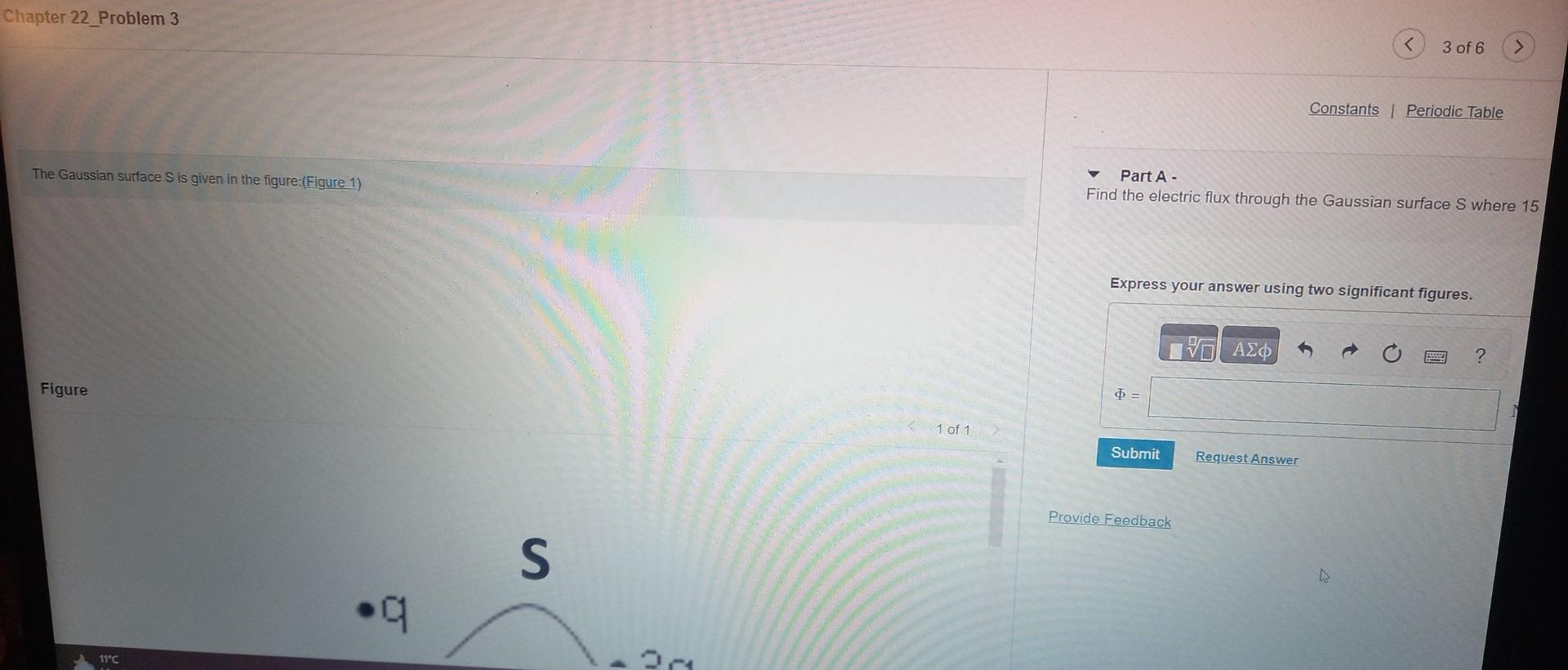# Question SThe Gaussian surface S is given in the figure:(Figure 1) Part A - Find the electric flux through the Gaussian surface S where 15 Express your answer using two significant figures. S The Gaussian surface <span class="katex"><span class="katex-html" aria-hidden="true"><span class="base"><span class="strut" style="height:0.6833em;"></span><span class="mord mathnormal" style="margin-right:0.05764em;">S</span></span></span></span> is given in the figure:(Figure 1) Part A - Find the electric flux through the Gaussian surface S where 15 Express your answer using two significant figures.electric flux through the Gaussian surface s where 15 ?
express the awnser using two significant figures!??

the Gaussian surface s is given in the figure 1

Transcribed Image Text: S The Gaussian surface is given in the figure:(Figure 1) Part A - Find the electric flux through the Gaussian surface S where 15 Express your answer using two significant figures.
More
Transcribed Image Text: S The Gaussian surface is given in the figure:(Figure 1) Part A - Find the electric flux through the Gaussian surface S where 15 Express your answer using two significant figures.&#12304;General guidance&#12305;The answer provided below has been developed in a clear step by step manner.Step1/2Answer : electric flux through the Gaussian surface S is given by the formula : electric flux ($$\mathrm{\phi}$$) = total charge enclosed in the gaussain surface / $$\mathrm{\epsilon}$$0 Explanation:this is the electric flux formulaExplanation:Please refer to solution in this step.Step2/2 electric flux ($$\mathrm{\phi}$$) = (q1 $$\mathrm{+}$$ q2$$\mathrm{+}$$ q3 \( \mathrm{+ ... See the full answer